x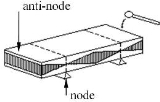Impulse excitation techniqueEncyclopedia

## Practical

The impulse excitation technique is a nondestructive test method that uses natural frequency, dimensions and mass of a test-piece to determine Young's modulus
Young's modulus
Young's modulus is a measure of the stiffness of an elastic material and is a quantity used to characterize materials. It is defined as the ratio of the uniaxial stress over the uniaxial strain in the range of stress in which Hooke's Law holds. In solid mechanics, the slope of the stress-strain...

, Shear modulus, Poisson's ratio
Poisson's ratio
Poisson's ratio , named after Siméon Poisson, is the ratio, when a sample object is stretched, of the contraction or transverse strain , to the extension or axial strain ....

and damping
Damping
In physics, damping is any effect that tends to reduce the amplitude of oscillations in an oscillatory system, particularly the harmonic oscillator.In mechanics, friction is one such damping effect...

coefficient.
Dimensions and mass of a test-piece can be easily measured. Natural frequency is determined by gently tapping the test-piece and analyzing the vibration. Tapping can be done using a small hammer or an automated tapping device. There are different ways to detect vibrations in the test-piece: piezoelectric sensor
Piezoelectric sensor
A piezoelectric sensor is a device that uses the piezoelectric effect to measure pressure, acceleration, strain or force by converting them to an electrical charge.-Applications:...

, microphone
Microphone
A microphone is an acoustic-to-electric transducer or sensor that converts sound into an electrical signal. In 1877, Emile Berliner invented the first microphone used as a telephone voice transmitter...

, laservibrometer or accelerometer
Accelerometer
An accelerometer is a device that measures proper acceleration, also called the four-acceleration. This is not necessarily the same as the coordinate acceleration , but is rather the type of acceleration associated with the phenomenon of weight experienced by a test mass that resides in the frame...

. To optimize the results a microphone or a laservibrometer can be used as there is no contact between the test-piece and the sensor. Laservibrometers are preferred to measure signals with extreme frequencies. These kinds of signals are usually only induced in very thin test-pieces.

### Flexure mode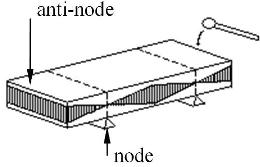This figure gives an example of a test-piece vibrating in the flexure
mode. The induced vibration is also referred to as the out-of plane vibration. The in-plane vibration is the vibration vibrating vertically on the out-of plane vibration. The natural frequency of this vibration is measured to calculate Young's modulus
Young's modulus
Young's modulus is a measure of the stiffness of an elastic material and is a quantity used to characterize materials. It is defined as the ratio of the uniaxial stress over the uniaxial strain in the range of stress in which Hooke's Law holds. In solid mechanics, the slope of the stress-strain...

.
To damp the test-piece as little as possible it has to be supported at the nodes. The test-piece is mechanically excited at one of the anti-nodes to cause maximum vibration.

### Torsion mode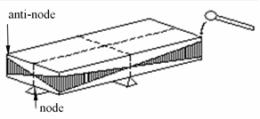This figure gives an example of a test-piece vibrating in the torsion
Torsion
The word torsion may refer to the following:*In geometry:** Torsion of a curve** Torsion tensor in differential geometry** The closely related concepts of Reidemeister torsion and analytic torsion ** Whitehead torsion*In algebra:** Torsion ** Tor functor* In medicine:** Ovarian...

mode. The natural frequency of this vibration is measured to calculate the Shear modulus.
To damp the test-piece as little as possible it has to be supported in the nodes. The test-piece is mechanically excited at one of the anti-nodes to cause maximum vibration.

### Signal conditioning and calculating result

After the signal is induced it has to be amplified and filtered. The resulting signal can than be introduced in a software package where it is logged. Software packages use algorithms to calculate Young's modulus
Young's modulus
Young's modulus is a measure of the stiffness of an elastic material and is a quantity used to characterize materials. It is defined as the ratio of the uniaxial stress over the uniaxial strain in the range of stress in which Hooke's Law holds. In solid mechanics, the slope of the stress-strain...

, Shear modulus, Poisson's ratio
Poisson's ratio
Poisson's ratio , named after Siméon Poisson, is the ratio, when a sample object is stretched, of the contraction or transverse strain , to the extension or axial strain ....

and damping
Damping
In physics, damping is any effect that tends to reduce the amplitude of oscillations in an oscillatory system, particularly the harmonic oscillator.In mechanics, friction is one such damping effect...

coefficient. The basic formulas used in these algorithms are discussed in the theory section.
Most software packages visualize the signal and show a graphic of the obtained frequencies.

### Area of application

There is a wide range of untested applications in which impulse excitation techniques (IET) could be used. Today IET is mostly used in research and quality control
Quality control
Quality control, or QC for short, is a process by which entities review the quality of all factors involved in production. This approach places an emphasis on three aspects:...

.
The advantage of IET in research projects is that it gives an in depth look into the internal structure of materials. Physical properties like damping
Damping
In physics, damping is any effect that tends to reduce the amplitude of oscillations in an oscillatory system, particularly the harmonic oscillator.In mechanics, friction is one such damping effect...

and Young's modulus
Young's modulus
Young's modulus is a measure of the stiffness of an elastic material and is a quantity used to characterize materials. It is defined as the ratio of the uniaxial stress over the uniaxial strain in the range of stress in which Hooke's Law holds. In solid mechanics, the slope of the stress-strain...

can be used for example to map out thermal shock
Thermal shock
Thermal shock is the name given to cracking as a result of rapid temperature change. Glass and ceramic objects are particularly vulnerable to this form of failure, due to their low toughness, low thermal conductivity, and high thermal expansion coefficients...

behaviour of certain materials.
Some machines even have the possibility to do measurements at very high temperatures, up to 1750 °C, or in vacuum
Vacuum
In everyday usage, vacuum is a volume of space that is essentially empty of matter, such that its gaseous pressure is much less than atmospheric pressure. The word comes from the Latin term for "empty". A perfect vacuum would be one with no particles in it at all, which is impossible to achieve in...

conditions. This can be an advantage in understanding the physical properties of certain materials.
Applications in quality control work differently. Most of these applications need a reference piece they can relate to. Engine blocks for example can be tested by tapping them and comparing the recorded signal with a pre-recorded signal of another engine block of which there is certainty its properties are satisfactory.
From a material point of view most materials that have enough stiffness
Stiffness
Stiffness is the resistance of an elastic body to deformation by an applied force along a given degree of freedom when a set of loading points and boundary conditions are prescribed on the elastic body.-Calculations:...

to vibrate can be measured using IET.

#### Young’s modulus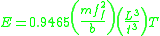Where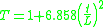E is Young's modulus
m is mass
ff is natural frequency in flexure dimension
b is width
L is length
t is thickness
The above formula can be used should L/t ≥ 20

#### Shear modulus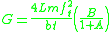Where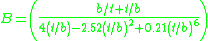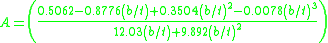Note we assume that b≥t

ft is the natural frequency in the torsion mode
m is mass
b is width
L is length
t is thickness

#### Young’s modulus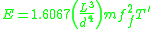Where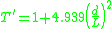E is Young's modulus
m is mass
ff is the natural frequency in flexure dimension
d is diameter
L is length
The above formula can be used should L/t ≥ 20

#### Shear modulus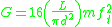Where
ft is the natural frequency in the torsion mode
m is mass
d is diameter
L is length

### Poisson ratio

If Young's modulus and Shear modulus are known Poisson's ratio can be calculated.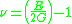### Damping coefficient

The signal induced in the test-piece is described as a damped sine
Sine
In mathematics, the sine function is a function of an angle. In a right triangle, sine gives the ratio of the length of the side opposite to an angle to the length of the hypotenuse.Sine is usually listed first amongst the trigonometric functions....

. The damping of this sine
Sine
In mathematics, the sine function is a function of an angle. In a right triangle, sine gives the ratio of the length of the side opposite to an angle to the length of the hypotenuse.Sine is usually listed first amongst the trigonometric functions....

can be determined by checking the force of consecutive amplitudes.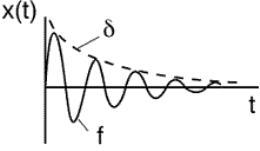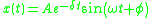Where
f = 1/T = ω/(2π) the natural frequency
δ = kt the logarithmic decrement
k the exponential damping of the vibration signal

## Accuracy

Accuracy is determined by the quality of support of the test-piece and the correctness of measurement of the test-piece’s dimensions and mass. It is possible to do measurements within an accuracy rate of 0,1%.
The best way to support a test-piece is to use nylon
Nylon
Nylon is a generic designation for a family of synthetic polymers known generically as polyamides, first produced on February 28, 1935, by Wallace Carothers at DuPont's research facility at the DuPont Experimental Station...

fibers, but most systems use polyurethane
Polyurethane
A polyurethane is any polymer composed of a chain of organic units joined by carbamate links. Polyurethane polymers are formed through step-growth polymerization, by reacting a monomer with another monomer in the presence of a catalyst.Polyurethanes are...

foam strips as support. This makes it very difficult to measure small test-pieces because the damping effect of the support is too big.

## Standards

• ASTM E 1875 Standard Test Method for Dynamic Young's Modulus, Shear Modulus, and Poisson's Ratio by Sonic Resonance
• ASTM E 1876 Standard Test Method for Dynamic Young's Modulus, Shear Modulus, and Poisson's Ratio by Impulse Excitation of Vibration